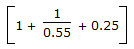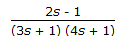# Chemical Engineering - Process Control and Instrumentation

### Exercise :: Process Control and Instrumentation - Section 3

16.

An ideal PID controller has the transfer function. The frequency at which the magnitude ratio of the controller is 1, is

 A. 0.5/0.2 B. 0.2/0.5 C. 0.2 x 0.5 D. 1/0.2 x 0.5

Explanation:

No answer description available for this question. Let us discuss.

17.

The deflection of the free end of the bimetallic strips in a bimetallic thermometer with temperature is nearly

 A. linear B. non-linear C. parabolic D. hyperbolic

Explanation:

No answer description available for this question. Let us discuss.

18.

Alphatron measures pressure __________ microns.

 A. < 1 B. < 3 C. > 3 D. < 0.1

Explanation:

No answer description available for this question. Let us discuss.

19.

What is the ratio of output amplitude to input amplitude for a sinusoidal forcing function in a first order system ?

 A. 1 B. >1 C. < 1 D. none of these

Explanation:

No answer description available for this question. Let us discuss.

20.

The unit step response of the transfer functionreaches its final steady state assymptotically after

 A. a monotonic increase. B. a monotonic decrease. C. initially increasing and then decreasing. D. increasing decreasing and then increasing.